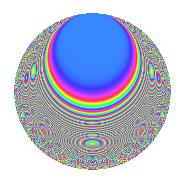# Properties

 Label 108.6.hLevel 108 Weight 6 Character orbit h Rep. character $$\chi_{108}(35,\cdot)$$ Character field $$\Q(\zeta_{6})$$ Dimension 56 Newform subspaces 1 Sturm bound 108 Trace bound 0

# Related objects

## Defining parameters

 Level: $$N$$ $$=$$ $$108 = 2^{2} \cdot 3^{3}$$ Weight: $$k$$ $$=$$ $$6$$ Character orbit: $$[\chi]$$ $$=$$ 108.h (of order $$6$$ and degree $$2$$) Character conductor: $$\operatorname{cond}(\chi)$$ $$=$$ $$36$$ Character field: $$\Q(\zeta_{6})$$ Newform subspaces: $$1$$ Sturm bound: $$108$$ Trace bound: $$0$$

## Dimensions

The following table gives the dimensions of various subspaces of $$M_{6}(108, [\chi])$$.

Total New Old
Modular forms 192 64 128
Cusp forms 168 56 112
Eisenstein series 24 8 16

## Trace form

 $$56q + 3q^{2} - q^{4} + 6q^{5} + O(q^{10})$$ $$56q + 3q^{2} - q^{4} + 6q^{5} - 68q^{10} - 2q^{13} + 1518q^{14} - q^{16} + 1242q^{20} + 63q^{22} + 12498q^{25} - 2052q^{28} + 11946q^{29} + 7233q^{32} + 6361q^{34} - 8q^{37} + 14877q^{38} - 1526q^{40} + 43536q^{41} - 26880q^{46} + 38414q^{49} - 38631q^{50} + 24988q^{52} - 21186q^{56} - 3314q^{58} - 2q^{61} - 106342q^{64} - 35970q^{65} - 31413q^{68} + 10524q^{70} + 53620q^{73} + 20406q^{74} + 26193q^{76} - 26178q^{77} - 151286q^{82} + 6248q^{85} - 279237q^{86} - 122541q^{88} - 435804q^{92} + 63480q^{94} - 58148q^{97} + O(q^{100})$$

## Decomposition of $$S_{6}^{\mathrm{new}}(108, [\chi])$$ into newform subspaces

Label Dim. $$A$$ Field CM Traces $q$-expansion
$$a_2$$ $$a_3$$ $$a_5$$ $$a_7$$
108.6.h.a $$56$$ $$17.321$$ None $$3$$ $$0$$ $$6$$ $$0$$

## Decomposition of $$S_{6}^{\mathrm{old}}(108, [\chi])$$ into lower level spaces

$$S_{6}^{\mathrm{old}}(108, [\chi]) \cong$$ $$S_{6}^{\mathrm{new}}(36, [\chi])$$$$^{\oplus 2}$$

## Hecke characteristic polynomials

There are no characteristic polynomials of Hecke operators in the database